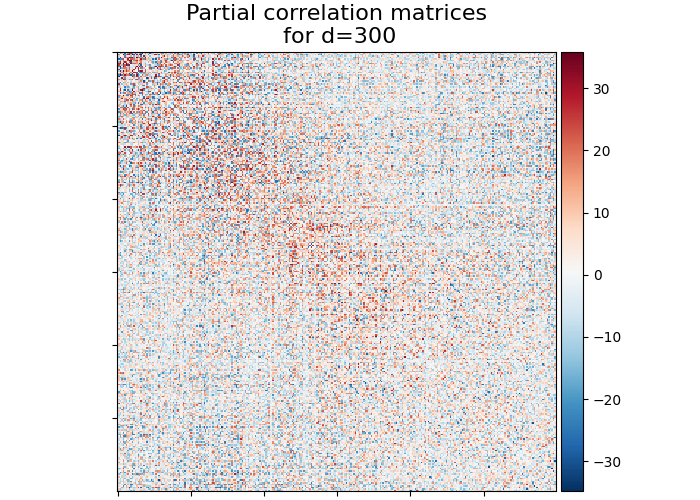# Visualizing Megatrawls Network Matrices from Human Connectome Project#

This example shows how to fetch network matrices data from HCP beta-release of the Functional Connectivity Megatrawl project.

See `nilearn.datasets.fetch_megatrawls_netmats` documentation for more details.

## Fetching the Megatrawls Network matrices#

Fetching the partial correlation matrices of dimensionality d=300 with timeseries method ‘eigen regression’

```from nilearn import datasets

netmats = datasets.fetch_megatrawls_netmats(
dimensionality=300,
timeseries="eigen_regression",
matrices="partial_correlation",
)
# Partial correlation matrices array of size (300, 300) are stored in the name
# of 'correlation_matrices'
partial_correlation = netmats.correlation_matrices
```

## Visualization#

Import nilearn plotting modules to use its utilities for plotting correlation matrices

```from nilearn import plotting

title = "Partial correlation matrices\n for d=300"
display = plotting.plot_matrix(partial_correlation, colorbar=True, title=title)
plotting.show()
```Total running time of the script: (0 minutes 0.546 seconds)

Estimated memory usage: 9 MB

Gallery generated by Sphinx-Gallery